"Penetrating Planets Since 2004"ECE6390 Introduction to Satellite Communications Fall, 2004
Initial ResearchIntroductionProject RequirementsNeptune FactsCalculations
Penetrating ProbesIntroductionDesignCalculationsParts & Pricing
Relay SatelliteIntroductionLink with ProbeCalculationsLink with EarthCalculationsParts & Pricing
ConclusionsSummaryTotal CostContact Info
Home » Initial Research » Calculations
 Calculations   The relay satellite in Geostationary Neptune Orbit (GNO) is the heart of the communications link between the probes and the Earth.  The satellite will act as a data gatherer and will transmit this data to the Earth whenever the satellite is in view of the Earth.  The satellite has been decided to be placed in equatorial orbit; the distance required for GNO is determined with the mass and period of Neptune: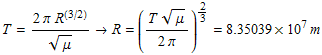The radius of GNO was calculated to be 83,503.9 km,  approximately three times the equatorial radius.  As a result of this large distance, the satellite will have to travel at 9.04706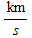in order to maintain its orbit and not be sucked in by Neptune's gravity.  Figure 1 shows a diagram of Neptune with a geostationary orbit.Figure 1: Geostationary Neptune Orbit              While the distance of free space between the satellite and the 0° probe can be calculated simply by subtracting the GNO radius and the equatorial radius, calculating the distance to the off-angle probes requires the use of the law of cosines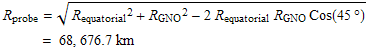The angle from the relay satellite to the off-angle probe can be determined byThis angle is important so that we can choose a dish antenna whose Half-Power Beamwidth (HPBW) will be close to approximately 30°.   The bit error rate (BER) determines the probability that a bit has incorrectly been received at the receiver that defines the quality of a digital communications system, much like the carrier to noise ratio in an analog system .  The statistical probability that a bit is incorrect often uses the Q-function, which is a "normalized form of the cumulative normal distribution function giving the probability that a variate assumes a value in the range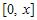" .  The Q-function is defined byFor an uncoded bi-polar phase shift keying (BPSK) signal, the BER is determined by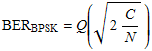whereis the carrier to noise ratio at the receiver in linear form.  Using a turbo code method of forward error correction essentially allows for an error-free communications link if the receivedis greater than about 1 or 2 dB (see Penetrating Probes » Design).     Next Section » References: 1. Pratt, Bostian, Alnutt. Satellite Communications, Second Edition. Copyright 2003. 2. Wolfram MathWorld (http://mathworld.wolfram.com/NormalDistributionFunction.html)
top of page

William W
e-mail